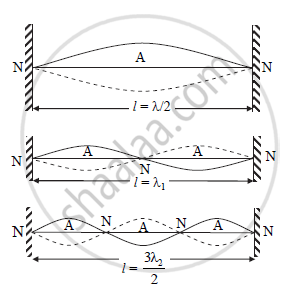HSC Science (General) 12th Board ExamMaharashtra State Board
Share

Show that all harmonics are present on a stretched string between two rigid supports - HSC Science (General) 12th Board Exam - Physics

ConceptStudy of Vibrations in a Finite Medium

Question

Show that all harmonics are present on a stretched string between two rigid supports.

SolutionVibration of string for different mode

Modes of vibrations in stretched string:-

a. Consider a string stretched between two rigid supports and plucked. Due to plucking, string vibrates and loops are formed in the string. Vibrations of string are as shown in figure.

b. Let,

p = number of loops

l = length of string

∴ Length of one loop = l /p                                                    ......(1)

c. Two successive nodes form a loop. Distance between two successive nodes is λ/2.

∴ Length of one loop = λ/2                                                     .......(2)

From equations (1) and (2),

λ/2 = l/p

∴ λ = 2l/p                                                                             ...........(3)

d. Velocity of transverse wave is given by,

v=sqrt(T/m)

e. Frequency of string is given by,

n = v/λ

Substituting λ from equation (3),

n=sqrt(T/m)/((2l)/p)

thereforen=p/(2l)sqrt(T/m)                                                                   .............(4)

f. Fundamental mode or first harmonic:-

In this case, p = 1

∴ From equation (4),

n=1/(2l)sqrt(T/m)

This frequency is called fundamental frequency.

g. First overtone or second harmonic:-

In this case, p = 2

∴ From equation (4),

n_1=2/(2l)sqrt(T/m)=2xx1/(2l)sqrt(T/m)=2n

thereforen_1=2n

h. Second overtone or third harmonic:-

In this case, p = 3

Using equation (4),

n_2=3/(2l)sqrt(T/m)=3xx1/(2l)sqrt(T/m)=3n

thereforen_2=3n

i. (p - 1)th overtone or pth harmonic:-

n_((p-1))=pxx1/(2l)sqrt(T/m)=pn

For pth overtone,

n_p=(p+1)/(2l)sqrt(T/m)=(p+1)n

j. Thus, in the vibration of stretched string, frequencies of vibrations are n, 2n, 3n,…..so on.

Hence, all harmonics (even as well as odd) are present in the vibrations of stretched string.

Is there an error in this question or solution?

APPEARS IN

2014-2015 (October) (with solutions)
Question 4.3 | 3.00 marks
Solution Show that all harmonics are present on a stretched string between two rigid supports Concept: Study of Vibrations in a Finite Medium.
S